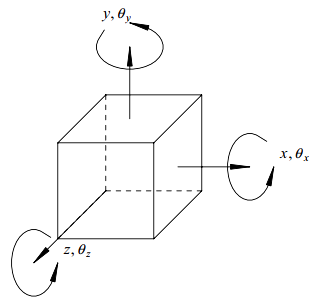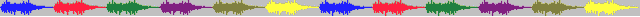# 1. 简介

《振动：解析与试验模态分析》 辛辛那提大学 Randall J. Allemang教授 (著)，KSI科尚仪器 董书伟 (译)

• 模态频率、特征值、系统极点、特征根$(\lambda _{r})$
• 模态向量、特征向量$(\left \{ \psi_{r},\,\phi_{r} \right \})$
• 模态系数$(\psi _{pr})$、留数$(A _{pqr})$
• 实模态向量、复模态向量
• 物理坐标、广义坐标
• 主坐标、模态坐标
• 模态质量、模态阻尼和模态刚度$(M _{r},\,C_{r},\,K_{r})$
• 模态$A$、模态$B\; (M_{A_r},\, M_{B_r})$

### 1.1 自由度### 1.2 基本假设《模态空间系列文章》Peter Avitabile教授(著) KSI科尚仪器 董书伟(译)…

《振动：试验模态分析》辛辛那提大学Randall J. Allemang教授(著) KSI科尚仪器 董书伟(译)…[new]